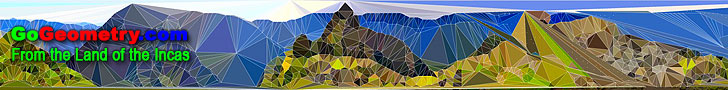# Welcome to GoGeometry: Recent additions (Page 6), Online LearningGo Geometry from the Land of the Incas - Recent Additions (Page 6 of 50)Geometry Problem 893 Triangle, Circumcircle, Incenter, Circle, Tangent, Collinear Points. GeoGebra, HTML5 Animation for Tablets Geometry Problem 892 Triangle, 60 degrees, Orthocenter, Circumcenter. Geometry Problem 890 Two Squares inscribed in Circles, Concurrent Lines. GeoGebra, HTML5 Animation for Tablets. Geometry Problem 889 Carnot's Theorem in an acute triangle, Circumcenter, Circumradius, Inradius. GeoGebra, HTML5 Animation for Tablets. Geometry Art 871 - 880. Congruent Circles, Intersecting Circles, Secant, Center, Angle Trisection, Triple Angle. Geometry Problem 888 Butterfly Theorem, Circle, Chord, Midpoint. GeoGebra, HTML5 Animation for Tablets. Geometry Problem 887 Triangle, Altitude, Angle Bisector, Perpendicular, Midpoint, Concyclic Points. GeoGebra, HTML5 Animation for Tablets. Sacred and Profane Love by Titian and Golden Rectangles, Droste EffectHTML5 Animation for Tablets, iPad and Nexus. Geometry Problem 886 Right Triangle, Incenter, Angle Bisector, Perpendicular, 45 Degrees, Concyclic Points, Isosceles Right Triangle. GeoGebra, HTML5 Animation for Tablets (iPad, Nexus). Geometry Problems 871 - 880. Triangle, Circle, Parallelogram, Trapezoid, Congruence, Similarity, Metric Relations, Incircle, Rectangle, Square Assumption of the Virgin by Titian and Golden Rectangles, Droste EffectHTML5 Animation for Tablets, iPad and Nexus. Geometry Art 851 - 860. Square, Semicircle, Quadrant, Arc, Perpendicular, Metric Relations Geometry Problem 885 Intersecting Circles, Three Tangent Lines, Midpoint. Geometry Problem 884: The Pythagorean Curiosity Right Triangles and squares, fifteen conclusions. Geometry Problem 883 Five Circumscribed Quadrilaterals, Circle, Tangent, Common Tangent. Geometry Problem 882 Triangle, Angle x, 2x, 7x, Cevian, Congruence, Auxiliary Lines. Bacchus and Ariadne by Titian and Golden Rectangles, Droste EffectHTML5 Animation for Tablets, iPad and Nexus. Geometry Problem 881 Triangle, three Squares, Centers, Areas. Geometry Problem 880 Triangle, Midpoints, Sides, Perpendiculars, Hexagon, Area. Geometry Problem 879 Square, Midpoint, Diagonal, Ratio 3:1, Angle Measure. Geometry Problem 878 Square, Inscribed Circle, Quadrant, Center, Perpendicular, Metric Relations. Geometry Problem 877 Square, Right Triangle, Similarity, Metric Relations, Transversal. Geometry Problem 876 Equilateral Triangle, any Point, Perpendicular, Right Triangle Area, Sum of Areas. Geometry Problem 875 Congruent Circles, Intersecting Circles, Secant, Center, Angle Trisection, Triple Angle. GeoGebra, Dynamic Geometry, Euclidean Egg with 8 Arcs in 5 Steps, Step-by-Step Construction HTML5 Animation for Tablets (iPad, Nexus) Geometry Problem 874 Isosceles Triangle, Circumcircle, Circumcenter, Congruence. Geometry Problem 873 Quadrilateral, Diagonal, Triangle, Angle Bisector, Congruence. Geometry Problems 851 - 860. Triangle, Circle, Square, Area, Parallel, Metric Relations, 30 Degrees, Congruence, Parallelogram Geometry Problems 861 - 870. Triangle, Circle, Parallelogram, Trapezoid, Congruence, Similarity, Metric Relations, Incircle, Rectangle, Square Flora (1515) by Titian and Golden Rectangles, Droste EffectHTML5 Animation for Tablets, iPad and Nexus. Salome (1515) by Titian and Golden Rectangles, Droste EffectHTML5 Animation for Tablets, iPad and Nexus. Geometry Art 861 - 870. Cyclic Quadrilateral, Triangle, Circle, Rectangle, Square, Center, 90 Degrees, Midpoint, Congruence Geometry Problem 872 Quadrilateral, Triangle, Angle, 30, 90 degrees. GeoGebra, Dynamic Geometry, Euclidean Egg, Step-by-Step Construction HTML5 Animation for Tablets (iPad, Nexus) Geometry Problem 871 Brahmagupta's Theorem, Cyclic Quadrilateral, Perpendicular Diagonals, Midpoint. White House Front, Washington D.C. and Golden Rectangles, Droste EffectHTML5 Animation for Tablets, iPad and Nexus. Geometry Problem 870 Triangle, Median, Three Squares, Centers, Vertices, Collinear Points, Midpoint. Geometry Problem 869 Triangle, Median, Three Equilateral Triangles, Collinear Points, Midpoint. The Taj Mahal and Golden Rectangles, Droste Effect, Fibonacci Spiral. HTML5 Animation for Tablets, iPad and Nexus. White House Front 2, Washington D.C. and Golden Rectangles, Droste EffectHTML5 Animation for Tablets, iPad and Nexus. GeoGebra, Dynamic Geometry: Internal Feuerbach Point HTML5 Animation for Tablets (iPad, Nexus..) Geometry Problem 868 Cyclic Quadrilateral, Circle, Five Rectangles, Four Centers, Congruence. Solly Madonna by Raphael and Golden Rectangles. HTML5 Animation for iPad and Nexus. Geometry Problem 867 Isosceles Triangle, Median, Perpendicular, Angle, Congruence. Geometry Problem 866 Cyclic Quadrilateral, Circle, Rectangle, Center, Congruence, 90 Degrees. Geometry Problem 865 Parallelogram, Diagonal, Midpoint, Side, Triangle, Parallel, Similarity. Geometry Problem 864 Parallelogram, Diagonal, Congruence, Similarity, Metric Relations. Circle Area. Index. Theorems and Problems. Go to Page: Previous | 1 | 2 | 3 | 4 | 5 | 6 | 7 | 8 | 9 | 10 | 11 | 12 | 13 | 14 | 15 | 16 | 17 | 18 | 19 | 20 | 21 | 22 | 23 | 24 | 25 | 26 | 27 | 28 | 29 | 30 | 31 | 32 | 33 | 34 | 35 | 36 | 37 | 38 | 39 | 40 | 41 | 42 | 43 | 44 | 45 | 46 | 47 | 48 | 49 | 50 | Next

 Home | GoGeometry Education | Geometry | Search | Email Last updated Aug 25, 2014